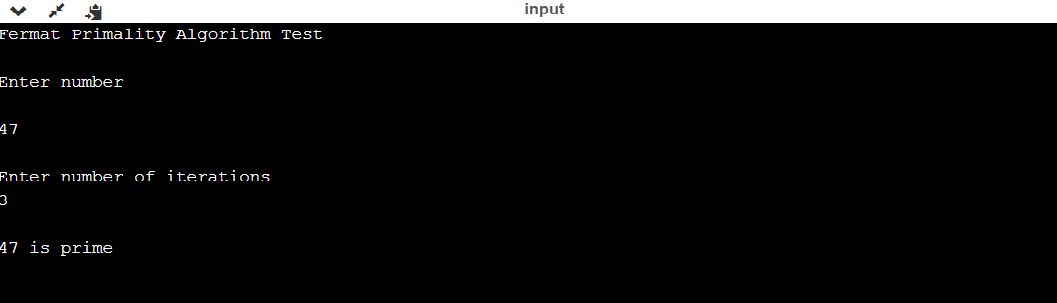Performing Baillie-PSW Primality Test in Java

• Last Updated : 06 May, 2021

The Baillie PSW Primality testing is generally a probabilistic primality testing Algorithm that tries to define whether a given number is composite or a probable prime, it has been given name after Robert Baillie. This test is proposed by Baillie and Wagstaff(1980) and Pomerance(1980 and 1984) which is based on Strong Pseudoprimes and Lucas Pseudoprimes. A big wow-fact is Pomerance originally declared a prize amount of \$30 for anyone who discovers a composite number that can pass this test and this prize amount was later raised to \$620. Interestingly, there have been no examples of composite numbers passing this test and as of June 13, 2009, Jeff Gilchrist had made an announcement that there are no Baillie-PSW pseudoprimes up to 10^(17). But however, the elliptic curve primality program PRIMO which checks all intermediate probable primes with this test, just in case if there were any probable composite numbers this certification would have failed and this never happened and PRIMO program author M. Martin estimated confidently that there is no composite less than about 10000 digits that can fool this test that existed.

Example:

Java

 // Java Program to implement Baillie - PSW Primality test// Importing utility classesimport java.util.Scanner;import java.util.Random;  // Class FermatPrimalitypublic class GFG {      // Method 1    // To check if prime or not    public boolean isPrime(long n, int iteration) {          // Base case        if (n == 0 || n == 1)            return false;          // Base case 2        //  is prime        if (n == 2)            return true;          // An even number other than 2 is composite        if (n % 2 == 0)            return false;          // Creating object of Random class        Random rand = new Random();        for (int i = 0; i < iteration; i++) {              // Getting positive valuee using absolute function            // of Math class            long r = Math.abs(rand.nextLong());            long a = r % (n - 1) + 1;            if (modPow(a, n - 1, n) != 1)                return false;        }        return true;    }      // Method 2    // To calculate (a ^ b) % c    public long modPow(long a, long b, long c) {          // Initially declaring and initializing the long variable        // to unity        long res = 1;        for (int i = 0; i < b; i++) {            res *= a;            res %= c;        }        return res % c;    }    // Method 3    // Main driver method    public static void main (String[] args) {          // Creating object of Scanner class to take input from user        Scanner scan = new Scanner(System.in);          // Display message only        System.out.println("Fermat Primality Algorithm Test\n");          // Make an object of GFG class        GFG fp = new GFG();          // Display message only        System.out.println("Enter number\n");          // Accepting the number using nextLong() method        long num = scan.nextLong();          // Display message only        System.out.println("\nEnter number of iterations");          // Accepting number of iterations using nextInt() method        int k = scan.nextInt();          // Check if prime        boolean prime = fp.isPrime(num, k);        if (prime)            System.out.println("\n" + num + " is prime");        else            System.out.println("\n" + num + " is composite");    }}

Output:Output Explanation:

Firstly, we construct a method isPrime() to find the given number is prime or not. We create a method modPow(), which is used for finding modulus to calculate logic for isPrime() method. We take input from user and apply the function isPrime(), as part of primality testing we take the number of iterations. We apply the methods isPrime() and findPow() for the number and given the number of iterations and generate the output after successfully conducting Baillie=PSW Primality Testing. We output the result whether the given number is prime or composite.

My Personal Notes arrow_drop_up